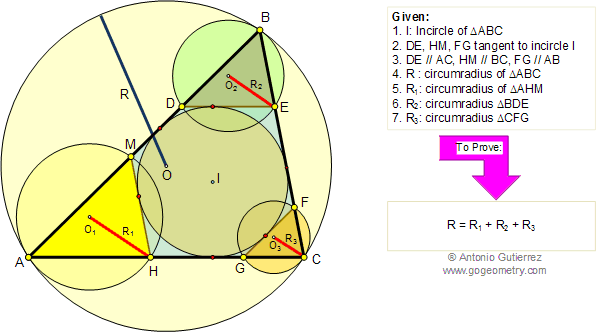Problem 143. Four Triangles, Incircle, Tangent and Parallel to Side, Circumradii.
 In the figure below, given a triangle ABC and the incircle of center I (inscribed circle), DE, FG, and HM are tangent to the incircle I and  parallel to AC, AB, and BC respectively. If R, R1, R2, R3 are the circumradii of triangles ABC, AHM, BDE, and CFG respectively, prove that R = R1 + R2 + R3. View or post a solution.Home | Geometry | Search | Wolfram Demonstrations Project | Problems | 141-150 | Email | By Antonio Gutierrez